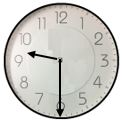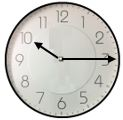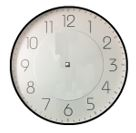## LetsPlayMaths.Com

WELCOME TO THE WORLD OF MATHEMATICS

### Class 2 - Annual Examination – 1

Objective Question:              [2X10=20]

1. A rectangle has ______ faces and ______ corners.

2. 1 fortnight = ______ days.

3. Which month comes before 6th month?

4. Which solid object has no corners and edges?

5. What time will be 2 hours before 1 o’ clock?

6. How many days are there in the month of July and August?

7. How many days are there in the month of February of a leap year?

8. If yesterday is Sunday then tomorrow will be ____________.

9. A triangle and a square together have ________ sides.

10. 41 x 65 = 65 x _______.

Objective Question:              [3X5=15]

11. Read the time and write it.12. Arrange the given shapes in increasing order according to the number of faces:
Cube, Cone, Cylinder, Sphere.

13. Draw the hands of the clock to show the given time.

3 O’ clock5:3014.
a) How many Rs. 2 coins will make Rs. 100?

b) Rs. 75 + Rs. 150 + Rs. 200 = __________.

15. A sweet stall sold 675 sweets in 5 days. How many sweet did it sell in each day?

Objective Question:              

16. There are 103 chocolates in a packet. Distribute all the chocolates equally among 5 boys. How many chocolates each boy will get and how many chocolates will remain?